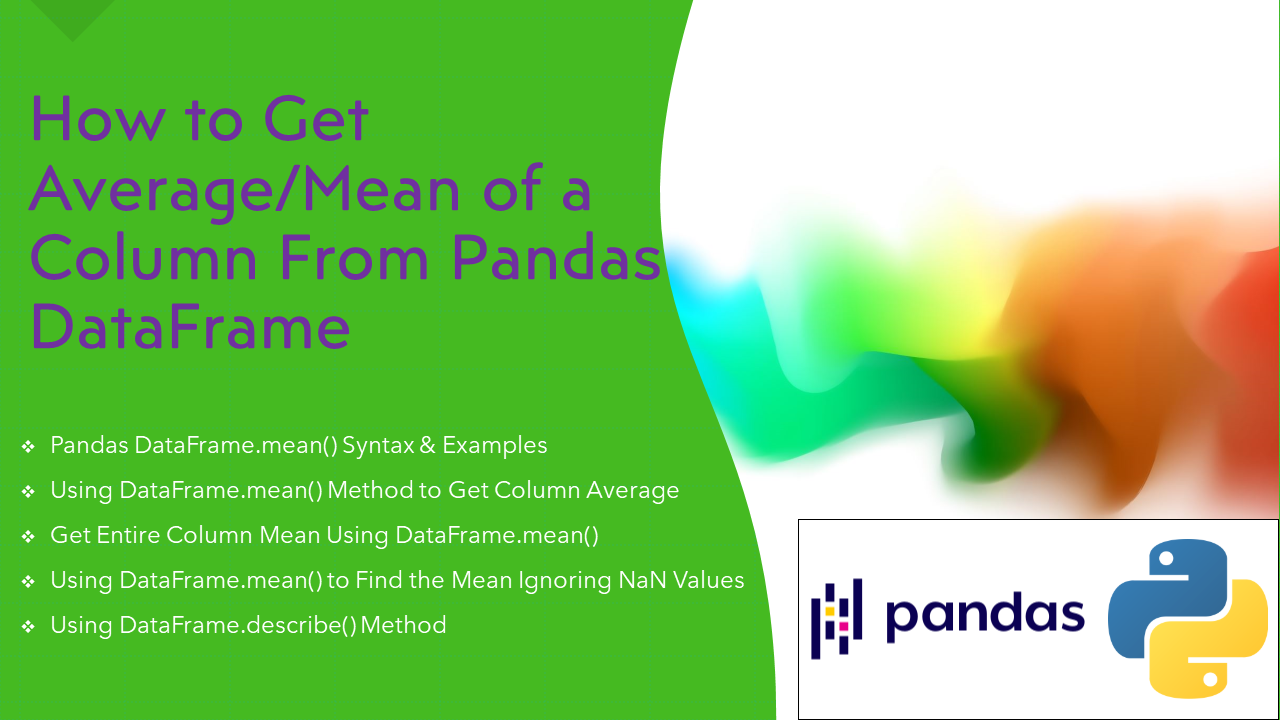# How to Get Column Average or Mean in pandas DataFrame

To get column average or mean from pandas DataFrame use either `mean()` and `describe()` method. The DataFrame.mean() method is used to return the mean of the values for the requested axis. If you apply this method on a series object, then it returns a scalar value, which is the mean value of all the observations in the pandas DataFrame.

In this article, I will explain how to get column average or mean from pandas DataFrame with examples.

## 1. Quick Examples of Get Column Average Or Mean In DataFrame

Below are some quick examples of how to get column average or mean in pandas DataFrame.

``````
# Below are quick example
# Using DataFrame.mean() method to get column average
df2 = df["Fee"].mean()

# Using DataFrame.mean() to get entire column mean
df2 = df.mean()

# Using multiple columns mean using DataFrame.mean()
df2 = df[["Fee","Discount"]].mean()

# Average of each column using DataFrame.mean()
df2 = df.mean(axis=0)

# Find the mean including NaN values using DataFrame.mean()
df2 = df.mean(axis = 0, skipna = False)

# Using DataFrame.describe() method
df2 = df.describe()
``````

Now, let’s create a DataFrame with a few rows and columns, execute these examples and validate results. Our DataFrame contains column names `Courses`, `Fee`, `Duration` and `Discount`.

``````
import pandas as pd
technologies = {
'Courses':["Spark","PySpark","Python","pandas",None],
'Fee' :[20000,25000,22000,None,30000],
'Duration':['30days','40days','35days','None','50days'],
'Discount':[1000,2300,1200,2000,None]
}
index_labels=['r1','r2','r3','r4','r5']
df = pd.DataFrame(technologies,index=index_labels)
print(df)
``````

Yields below output.

``````
Courses      Fee Duration  Discount
r1    Spark  20000.0   30days    1000.0
r2  PySpark  25000.0   40days    2300.0
r3   Python  22000.0   35days    1200.0
r4   pandas      NaN     None    2000.0
r5     None  30000.0   50days       NaN
``````

## 2. Get Column Mean

DataFrame.mean() method gets the mean value of a particular column from pandas DataFrame, you can use the` df["Fee"].mean() `function for a specific column only.

``````
# Using DataFrame.mean() method to get column average
df2 = df["Fee"].mean()
print(df2)
``````

Yields below output.

``````
24250.0
``````

## 4. Get Column Mean for All Columns

To calculate the mean of whole columns in the DataFrame, use `pandas.Series.mean()` with a list of DataFrame columns. You can also get the mean for all numeric columns using DataFrame.mean(), use `axis=0` argument to calculate the column-wise mean of the DataFrame.

``````
# Using DataFrame.mean() to get entire column mean
df2 = df.mean()
print(df2)

# Using multiple columns mean using DataFrame.mean()
df2 = df[["Fee","Discount"]].mean()
print(df2)

# Average of each column using DataFrame.mean()
df2 = df.mean(axis=0)
print(df2)
``````

Above all examples yields the same below output.

``````
Fee         24250.0
Discount     1625.0
dtype: float64
``````

## 5. Find the Mean Including NaN Values

By default mean() ignores/exclude NaN/null values while calculating mean or average, you can consider these values by using `skipna=False` param.

``````
# Find the mean ignoring NaN values using DataFrame.mean()
df2 = df.mean(axis = 0, skipna = False)
print(df2)
``````

I will leave it to you to execute this in your environment.

## 6. Using DataFrame.describe() Method

You can also use `DataFrame.describe()` to create the output of complete statistics of the data in DataFrame.

``````
# Using DataFrame.describe() method
df2 = df.describe()
print(df2)
``````

Yields below output.

``````
Fee     Discount
count      4.00000     4.000000
mean   24250.00000  1625.000000
std     4349.32945   623.832242
min    20000.00000  1000.000000
25%    21500.00000  1150.000000
50%    23500.00000  1600.000000
75%    26250.00000  2075.000000
max    30000.00000  2300.000000
``````

## 7. Complete Example For Get Column Average or Mean

``````
import pandas as pd
technologies = {
'Courses':["Spark","PySpark","Python","pandas",None],
'Fee' :[20000,25000,22000,None,30000],
'Duration':['30days','40days','35days','None','50days'],
'Discount':[1000,2300,1200,2000,None]
}
index_labels=['r1','r2','r3','r4','r5']
df = pd.DataFrame(technologies,index=index_labels)
print(df)

# Using DataFrame.mean() method to get column average
df2 = df["Fee"].mean()
print(df2)

# Using DataFrame.mean() to get entire column mean
df2 = df.mean()
print(df2)

# Using multiple columns mean using DataFrame.mean()
df2 = df[["Fee","Discount"]].mean()
print(df2)

# Average of each column using DataFrame.mean()
df2 = df.mean(axis=0)
print(df2)

# Find the mean ignoring NaN values using DataFrame.mean()
df2 = df.mean(axis = 0, skipna = False)
print(df2)

# Using DataFrame.describe() method
df2 = df.describe()
print(df2)
``````

### Conclusion

In this article, you have learned how to get column average or mean from pandas DataFrame using DataFrame.mean() and `DataFrame.describe()` method with examples. Using mean() you can get mean from single or selected columns and by index.

Happy Learning !!

### References